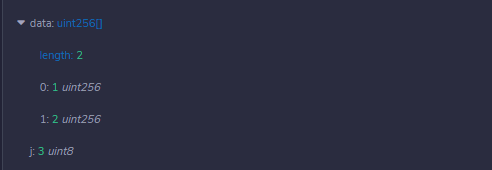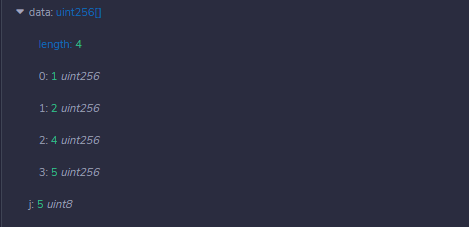# Solidity – Break and Continue Statements

• Last Updated : 11 May, 2022

In any programming language, Control statements are used to change the execution of the program. Solidity allows us to handle loops and switch statements. These statements are usually used when we have to terminate the loop without reaching the bottom or we have to skip some part of the block of code and start the new iteration. Solidity provides the following control statements to handle the program execution.

### Break Statement

This statement is used when we have to terminate the loop or switch statements or some block of code in which it is present. After the break statement, the control is passed to the statements present after the break. In nested loops, break statement terminates only those loops which has break statement.

Example: In the below example, the contract Types is defined consisting of a dynamic array, an unsigned integer variable, and a function containing the while loop to demonstrate the working of the break statement.

## Solidity

 `// Solidity program to demonstrate ` `// use of Break statement ` `pragma solidity ^0.5.0;  ` `  `  `// Creating a contract ` `contract` `Types {  ` `     `  `    ``// Declaring a dynamic array    ` `    ``uint[] data;  ` `   `  `    ``// Declaring the state variable ` `    ``uint8 j = 0; ` ` `  `    ``// Defining the function to  ` `    ``// demonstrate use of Break  ` `    ``// statement ` `    ``function loop( ` `    ``) ``public` `returns(uint[] memory){ ` `    ``while(j < 5) { ` `        ``j++; ` `        ``if``(j==3){ ` `            ``break``; ` `        ``} ` `        ``data.push(j); ` `     ``} ` `      ``return` `data; ` `    ``} ` `}`

Output :### Continue Statement

This statement is used when we have to skip the remaining block of code and start the next iteration of the loop immediately. After executing the continue statement, the control is transferred to the loop check condition, and if the condition is true the next iteration starts.

Example: In the below example, contract Types is defined consisting of a dynamic array, an unsigned integer variable, and a function containing the while loop to demonstrate the working of the continue statement.

## Solidity

 `// Solidity program to demonstrate ` `// use of Continue statement ` `pragma solidity ^0.5.0;  ` `  `  `// Creating a contract ` `contract` `Types {  ` `     `  `    ``// Declaring a dynamic array ` `    ``uint[] data;  ` `   `  `    ``// Declaring a state variable ` `    ``uint8 j = 0; ` ` `  `    ``// Defining a function to   ` `    ``// demonstrate the use of  ` `    ``// Continue statement ` `    ``function loop( ` `    ``) ``public` `returns(uint[] memory){ ` `    ``while(j < 5) { ` `        ``j++; ` `        ``if``(j==3){ ` `            ``continue``; ` `        ``} ` `        ``data.push(j); ` `     ``} ` `      ``return` `data; ` `    ``} ` `}`

Output :My Personal Notes arrow_drop_up
Recommended Articles
Page :